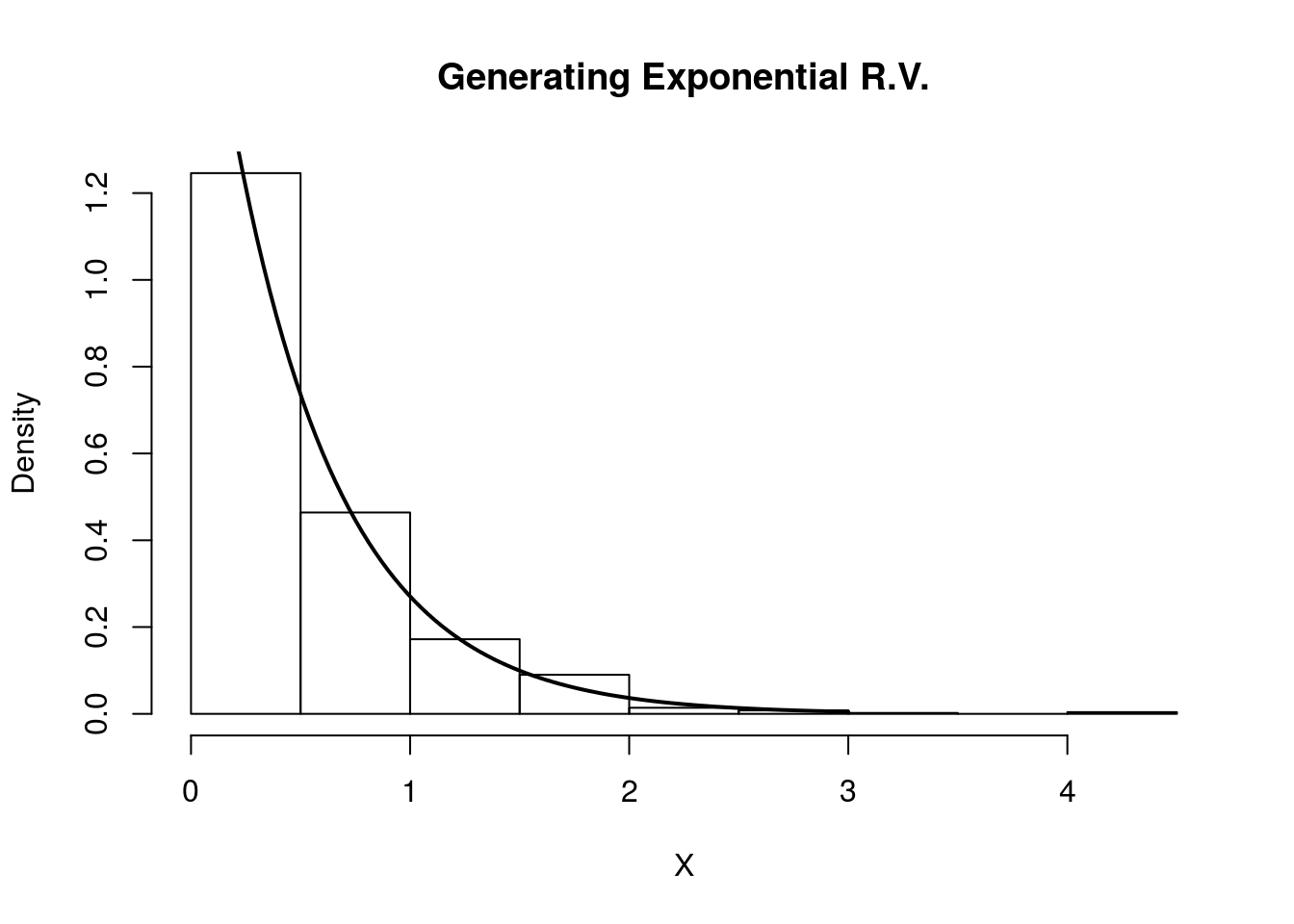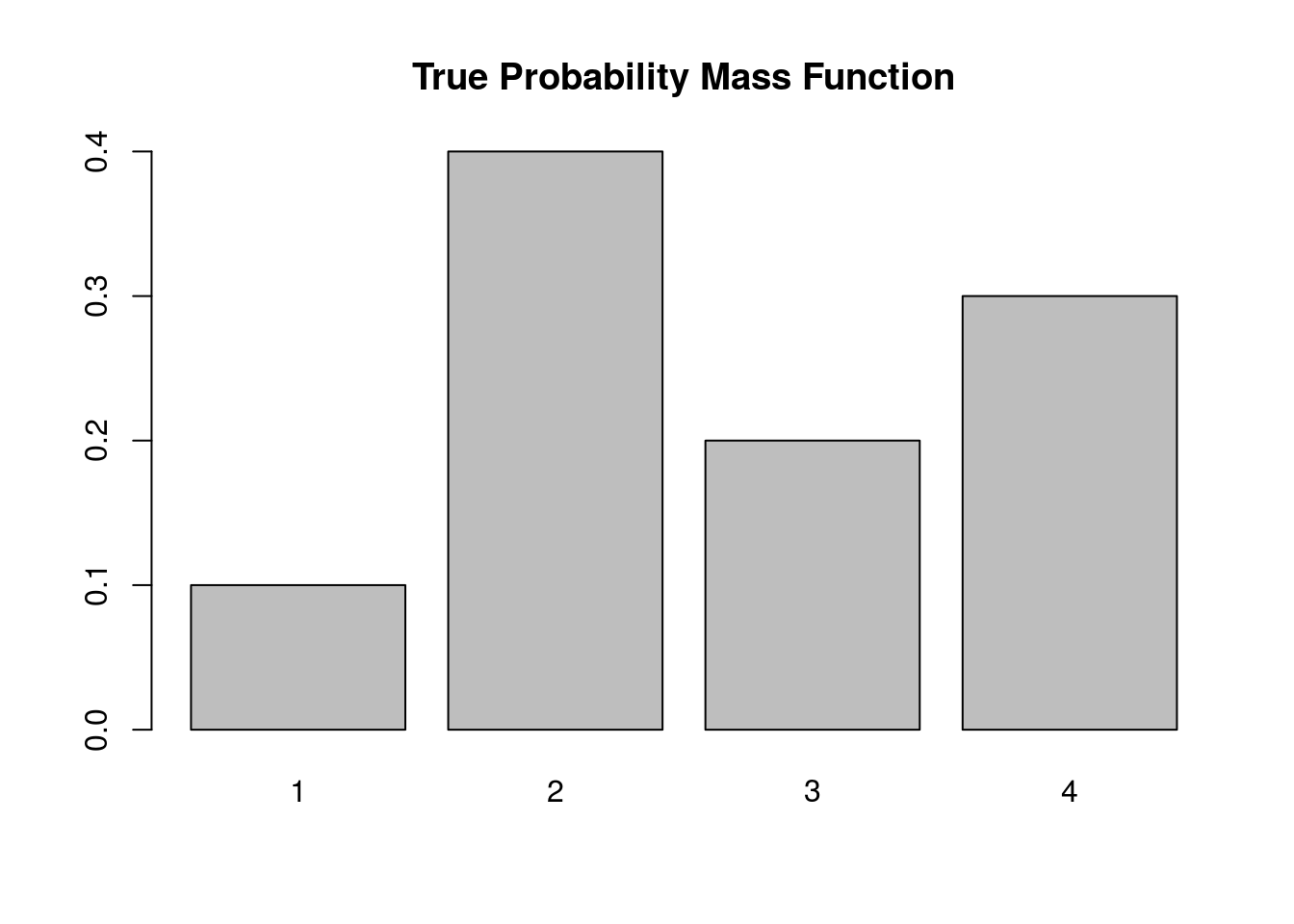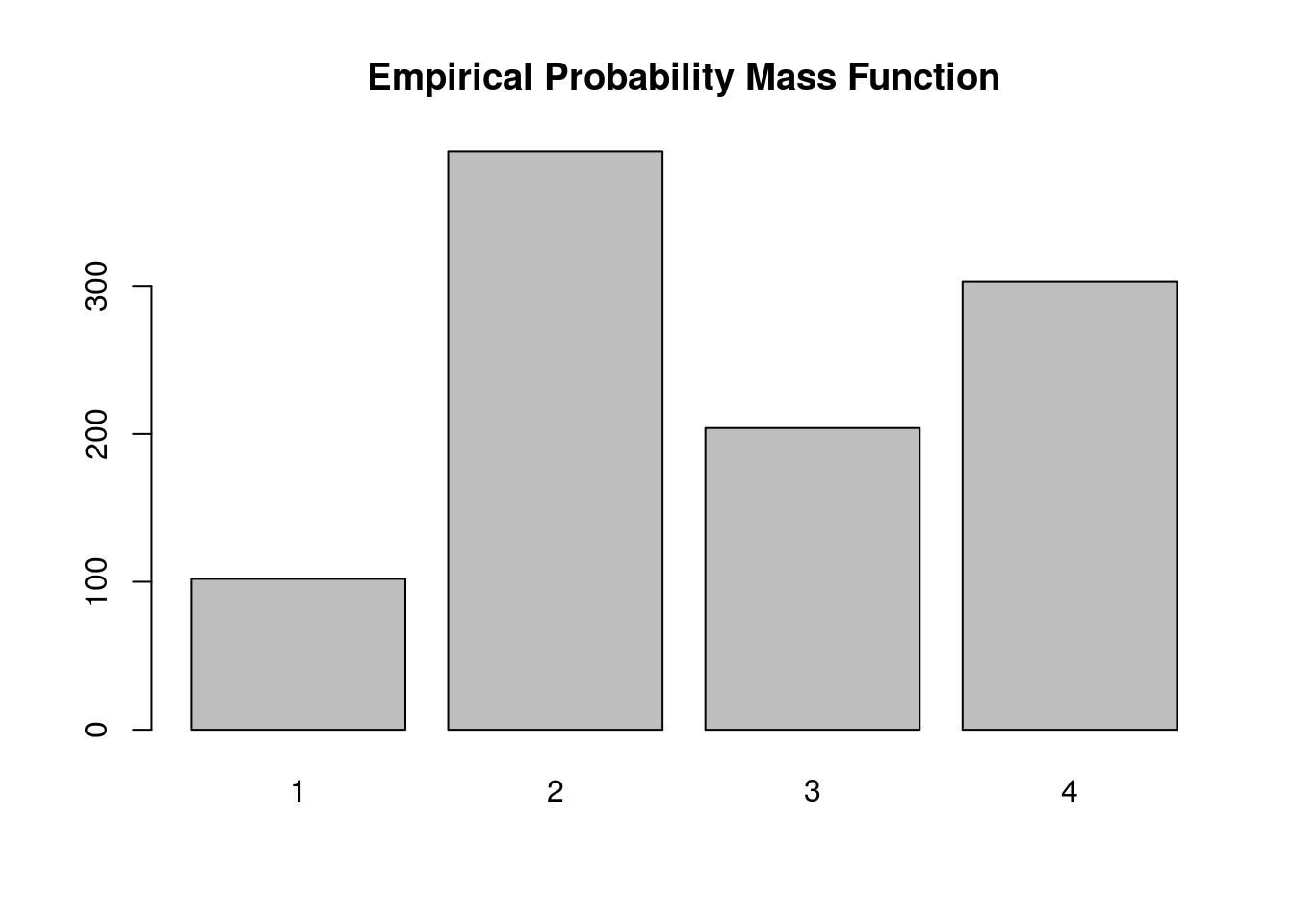Last updated: 2017-03-04

Code version: 5d0fa13282db4a97dc7d62e2d704e88a5afdb824

# Pre-requisites

This document assumes basic familiarity with probability theory.

# Overview

Inverse transform sampling is a method for generating random numbers from any probability distribution by using its inverse cumulative distribution $$F^{-1}(x)$$. Recall that the cumulative distribution for a random variable $$X$$ is $$F_X(x) = P(X \leq x)$$. In what follows, we assume that our computer can, on demand, generate independent realizations of a random variable $$U$$ uniformly distributed on $$[0,1]$$.

# Algorithm

## Continuous Distributions

Assume we want to generate a random variable $$X$$ with cumulative distribution function (CDF) $$F_X$$. The inverse transform sampling algorithm is simple:
1. Generate $$U \sim \text{Unif}(0,1)$$
2. Let $$X = F_X^{-1}(U)$$.

Then, $$X$$ will follow the distribution governed by the CDF $$F_X$$, which was our desired result.

Note that this algorithm works in general but is not always practical. For example, inverting $$F_X$$ is easy if $$X$$ is an exponential random variable, but its harder if $$X$$ is Normal random variable.

## Discrete Distributions

Now we will consider the discrete version of the inverse transform method. Assume that $$X$$ is a discrete random variable such that $$P(X = x_i) = p_i$$. The algorithm proceeds as follows:
1. Generate $$U \sim \text{Unif}(0,1)$$
2. Determine the index $$k$$ such that $$\sum_{j=1}^{k-1} p_j \leq U < \sum_{j=1}^k p_j$$, and return $$X = x_k$$.

Notice that the second step requires a search.

Assume our random variable $$X$$ can take on any one of $$K$$ values with probabilities $$\{p_1, \ldots, p_K\}$$. We implement the algorithm below, assuming these probabilities are stored in a vector called p.vec.

# note: this inefficient implementation is for pedagogical purposes
# in general, consider using the rmultinom() function
discrete.inv.transform.sample <- function( p.vec ) {
U  <- runif(1)
if(U <= p.vec){
return(1)
}
for(state in 2:length(p.vec)) {
if(sum(p.vec[1:(state-1)]) < U && U <= sum(p.vec[1:state]) ) {
return(state)
}
}
}

# Continuous Example: Exponential Distribution

Assume $$Y$$ is an exponential random variable with rate parameter $$\lambda = 2$$. Recall that the probability density function is $$p(y) = 2e^{-2y}$$, for $$y > 0$$. First, we compute the CDF: $F_Y(x) = P(Y\leq x) = \int_0^x 2e^{-2y} dy = 1 - e^{-2x}$

Solving for the inverse CDF, we get that $F_Y^{-1}(y) = -\frac{\ln(1-y)}{2}$

Using our algorithm above, we first generate $$U \sim \text{Unif}(0,1)$$, then set $$X = F_Y^{-1}(U) = -\frac{\ln(1-U)}{2}$$. We do this in the R code below and compare the histogram of our samples with the true density of $$Y$$.

# inverse transfrom sampling
num.samples <-  1000
U           <-  runif(num.samples)
X           <- -log(1-U)/2

# plot
hist(X, freq=F, xlab='X', main='Generating Exponential R.V.')
curve(dexp(x, rate=2) , 0, 3, lwd=2, xlab = "", ylab = "", add = T)Indeed, the plot indicates that our random variables are following the intended distribution.

# Discrete Example

Let’s assume we want to simulate a discrete random variable $$X$$ that follows the following distribution:

x_i P(X=x_i)
1 0.1
2 0.4
3 0.2
4 0.3

Below we simulate from this distribution using the discrete.inv.transform.sample() function above, and plot both the true probability vector, and the empirical proportions from our simulation.

num.samples <- 1000
p.vec        <- c(0.1, 0.4, 0.2, 0.3)
names(p.vec) <- 1:4
samples     <- numeric(num.samples)
for(i in seq_len(num.samples) ) {
samples[i] <- discrete.inv.transform.sample(p.vec)
}
barplot(p.vec, main='True Probability Mass Function')barplot(table(samples), main='Empirical Probability Mass Function')Again, the plot supports our claim that we are drawing from the true probability distribution

# Session information

sessionInfo()
R version 3.3.0 (2016-05-03)
Platform: x86_64-apple-darwin13.4.0 (64-bit)
Running under: OS X 10.10.5 (Yosemite)

locale:
 en_NZ.UTF-8/en_NZ.UTF-8/en_NZ.UTF-8/C/en_NZ.UTF-8/en_NZ.UTF-8

attached base packages:
 stats     graphics  grDevices utils     datasets  methods   base

other attached packages:
 tidyr_0.4.1     dplyr_0.5.0     ggplot2_2.1.0   knitr_1.15.1
 MASS_7.3-45     expm_0.999-0    Matrix_1.2-6    viridis_0.3.4
 workflowr_0.3.0 rmarkdown_1.3

loaded via a namespace (and not attached):
 Rcpp_0.12.5      git2r_0.18.0     plyr_1.8.4       tools_3.3.0
 digest_0.6.9     evaluate_0.10    tibble_1.1       gtable_0.2.0
 lattice_0.20-33  shiny_0.13.2     DBI_0.4-1        yaml_2.1.14
 gridExtra_2.2.1  stringr_1.2.0    gtools_3.5.0     rprojroot_1.2
 grid_3.3.0       R6_2.1.2         reshape2_1.4.1   magrittr_1.5
 backports_1.0.5  scales_0.4.0     htmltools_0.3.5  assertthat_0.1
 mime_0.5         colorspace_1.2-6 xtable_1.8-2     httpuv_1.3.3
 labeling_0.3     stringi_1.1.2    munsell_0.4.3   

This site was created with R Markdown